08-401/On the Term Test

(diff) ← Older revision | Latest revision (diff) | Newer revision → (diff)
Announcements go here

Our Term Test will take place on February 27 at 6:20PM at Galbraith (GB) 304 on 35 St. George Street, across from the Bahen Centre for Information Technology. It will be two hours long.

The material is everything covered in class until and including the class of February 13, 2007. Everything in the test be taken from our text book, and there will be two types of questions (or maybe sometimes the two types will be mixed within a single question):

• You may be asked to prove a theorem proven in class. The reason we prove theorems in class is that these proofs are important. Therefore I expect you to know them.
• You may be asked to solve exercises from the relevant chapters of the book, or minor variations thereof. These may be questions that were assigned as homework, but also, these may be questions that were not assigned before.

Office Hours. Dror will hold extended office hours on the week of the Term Test, on Monday from 2PM to 4PM, on Tuesday at the usual time (12:30-1:30) and on Wednesday from 1:30PM to 3PM, all at or near Bahen 6178. Yichao Zhang will hold his usual office hours, on Tuesday 1:00-3:00 at the Math Aid Centre, Sidney Smith 1071.

Preparing for the Test. Read, reread and rereread everything and solve lots of exercises from the book.

My (Dror's) system when I was an undergrad was to prepare a 2-3 page 50-100 item list of points covered in class. I'd only summarize each point with one sentence, without giving any details and without trying to be precise, much like the list below that I prepared for the class of February 6. I would then go over my list again and again and again, crossing out every item for which I was sure I could complete all the details and supply all the proofs. I would only stop when there was nothing left to cross out.

Good Luck!

Summary of the class of February 6:

 A long division computation.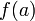$f(a)$ is the remainder of the division of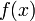$f(x)$ by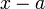$x-a$.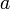$a$ is a zero of$f(x)$ iff$x-a$ is a factor of$f(x)$. The multiplicity of a zero. A polynomial of degree$n$ has at most$n$ roots, counting multiplicities. The roots of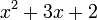$x^2+3x+2$ in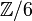${\mathbb Z}/6$. The roots of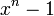$x^n-1$. Definition. A Principle Ideal Domain (PID).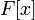$F[x]$ is a PID and a criterion for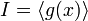$I=\langle g(x)\rangle$. Example. the complex numbers and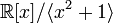${\mathbb R}[x]/\langle x^2+1\rangle$. Definition. Units in a ring. Definition. Reducible and irreducible polynomials. Reducibility in degrees 2 and 3. Primitive polynomials. The product of primitive polynomials is primitive. The content of a polynomial. The content of a product is the product of the contents. If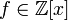$f\in{\mathbb Z}[x]$ is reducible in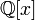${\mathbb Q}[x]$, it is reducible already in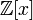${\mathbb Z}[x]$.